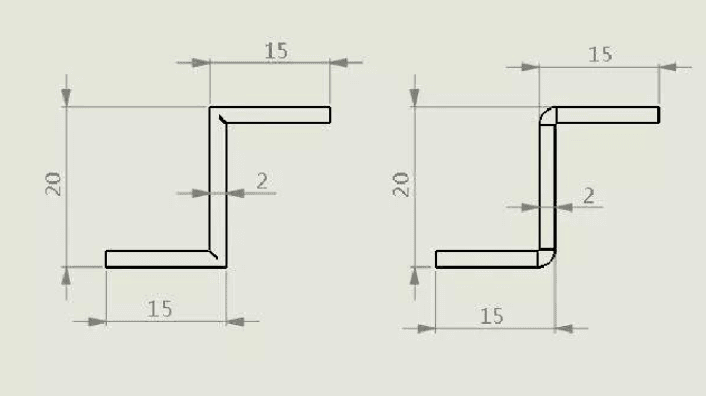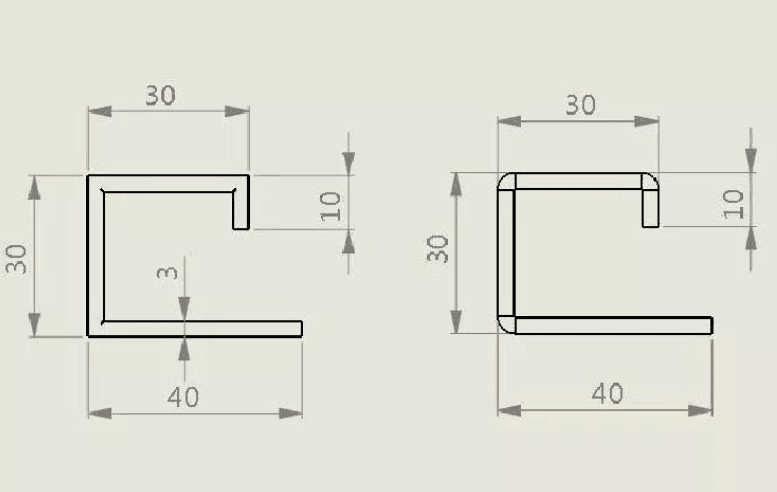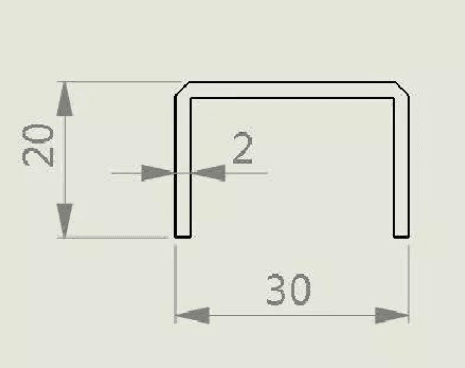Hi, how can I help?

# Calculation Method of V Grooving Machine Bending

With the continuous optimization and improvement of metal plate processing technology, the role of the V grooving machine is becoming more and more important. KRRASS in the sheet metal industry, with solid technical strength, exquisite production technology, strict quality management system, over the years specialized in the production of V grooving machine. We not only provide high-quality v groover machines but also offer v groove cutting machine information. Today, we will introduce the calculation method of the V grooving machine bending.

As shown in the figure, now use the groove, bend a 20×20 square tube, the plate thickness is 1mm.The thickness of the remaining board of the planning groove is related to the size of the right-angle arc after the customer bending, and is also related to the size of the plate thickness T. Generally speaking, when the plate thickness is less than or equal to 1mm, a=0.4mm; when the plate thickness is greater than 1mm, a= t /2.

t=2x(L1+L2)=2x ((length of side-1xa-0.2) + (length of side-2xa))=2x ((20-1×0.4-0.2) + (20-2×0.4))= 77.2mm

Note: one end of the L1 is grooved, no matter how thick the material, is deducted 0.2 mm the following through grooved bending and non-groove bending to compare the calculation of bending expansion difference:For grooved bending, the total length is calculated as follows: L=20-0.4+ 20-2×0.4 +20-0.4=58.4, for non-grooved bending, the total length is calculated as follows:

L= (20-1xt+ k) + (20-2xt+2xk) + (20-1xt+1xk) = (20-1+0.2) + (20-2+2×0.2) + (20-1+0.2) =56.8mm ,t is the thickness of the material, and k is the coefficient. The size of K is related to the thickness of the material, generally between 0.2-0.25. The thicker the material, the greater the value of k. Choose k for this example.

The following is to calculate the length of bending expansion by combining a Z-shaped groove with and without groove:For 2mm plate, plane groove residual material thickness a take t / 2 = 1 mm, (if the customer requests directly arc smaller, will have to choose a value less than 1 mm) and so on length: L = 15-1 + 20-2 x1 +15-1 = 46 mm, to 2 mm thick plate, not bending plane groove, choose 0.25 coefficient k and so on length: L = (15-2 + 0.25) + (20-2×2 + 2×0.25) + (15-2 + 0.25) = 43 mm under the combination of more than a bending workpiece groove and not groove example to introduce calculation method:For a 3mm thick plate, it is assumed that the customer requires a small arc at a right Angle. At this time, select 0.5mm for the remaining material thickness, and L= (40-0.5) + (30-2×0.5) + (30-2×0.5) + (10-0.5) =107mm;

For a 3mm thick plate, the coefficient K is 0.25, so the expansion length: L= (40-3+0.25) + (30-6+2×0.25) + (30-6+2×0.25) + (10-3+0.25) =93.5mm. The following is an example of reverse bending after grooving:For the post-planing reflexion as shown in the figure, the calculated expansion length is L= (20-2+0.2) + (30-2×2+2×0.2) + (20-2+0.2) =62.8mm